# Program to print interesting pattern

Solving interesting pattern problems enhances the understanding of loops. They are essential because they help in building a strong foundation of a particular programming language. There are various kinds of patterns including number-based, star-based and alphabetical patterns as well. In this article, we will discuss a few Java programs to print interesting star patterns.

## Program to Print Interesting Pattern

### Pattern 1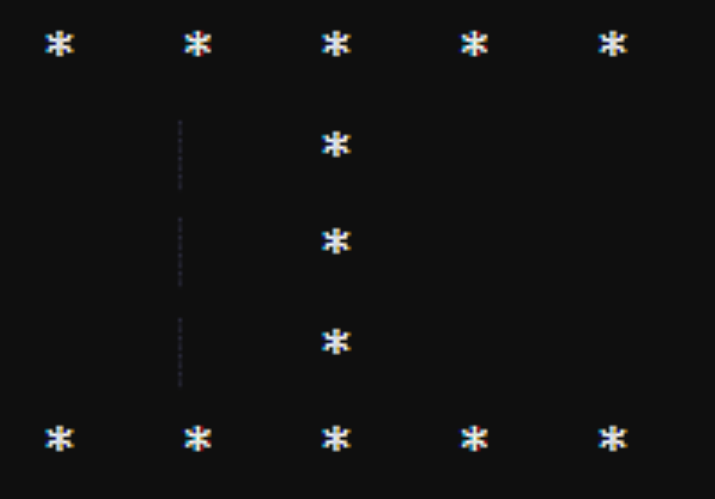### Approach

• Declare and initialize an integer ‘n’ that specifies number of rows and columns.

• Define a for loop that will run till ‘n’. Inside this loop define an if-else block.

• The if block will print star ‘n’ times in 1st and last row and else block will print the space ‘n/2’ means 2 times and star one time in 2nd to 4th row.

### Example

public class P1 {
public static void main(String[] args) {
int n = 5;
for(int i = 1; i <= n; i++) {
// till number of rows
if(i == 1 || i == n) {
for(int str = 1; str <= n; str++) {
System.out.print("*\t");
}
} else {
for(int spc = 1; spc <= n/2; spc++) {
System.out.print("\t");
}
for(int str = 1; str <= 1; str++){
System.out.print("*");
}
}
System.out.println();
// to move cursor to next line
}
}
}


### Output

*	*	*	*	*
*
*
*
*	*	*	*	*


### Pattern 2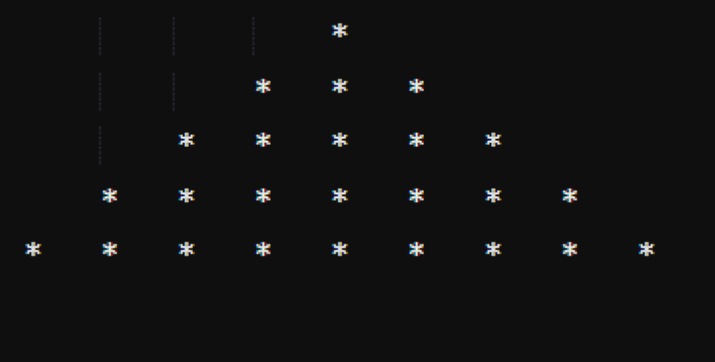### Approach

• Declare and initialize an integer ‘n’ that specifies number of rows.

• Create a nested for loop, the outer one will run till ‘n’ and inner for loop will run till number of spaces and print the spaces. After printing, we will decrement the space count by 1.

• Again take another inner for loop that will run till number of stars and print the stars. After printing, we will increment the star count by 2.

### Example

public class P2 {
public static void main(String[] args) {
int n = 5;
int spc = n-1; // initial space count
int str = 1; // initial star count
for(int i = 1; i <= n; i++) {
for(int j = 1; j <= spc; j++) { // spaces
System.out.print("\t");
}
spc--;
for(int k = 1; k <= str; k++) { // stars
System.out.print("*\t");
}
str += 2;
System.out.println();
}
}
}


### Output

				*
*	*	*
*	*	*	*	*
*	*	*	*	*	*	*
*	*	*	*	*	*	*	*	*


### Pattern 3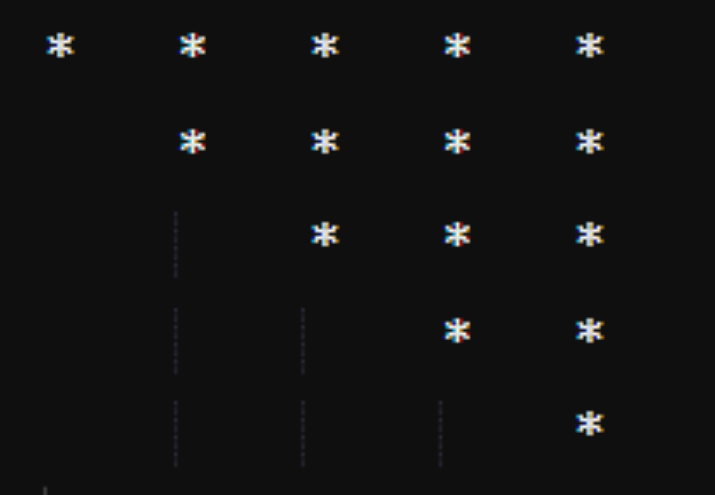### Approach

• Declare and initialize an integer ‘n’ that specifies number of rows.

• Define nested for loop, the outer one will run from ‘n’ to 1 and the first inner loop will print the space and the second one will print the stars.

### Example

public class P3 {
public static void main(String[] args) {
int n=5;
for(int i = n; i >= 1; i--) {
for(int spc = n-i; spc >= 1; spc--){ // spaces
System.out.print("\t");
}
for(int str = 1; str <= i; str++){ // stars
System.out.print("*\t");
}
System.out.println();
}
}
}


### Output

*	*	*	*	*
*	*	*	*
*	*	*
*	*
*


### Pattern 4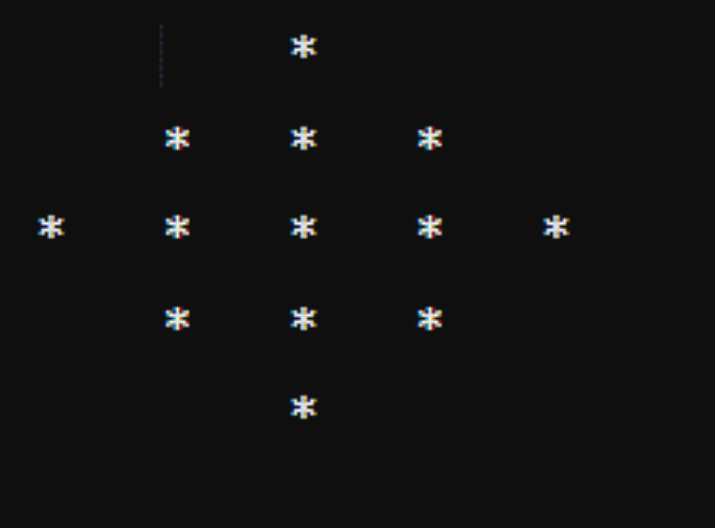### Approach

• Declare and initialize an integer ‘n’ that specifies number of rows.

• Create a nested for loop, the outer one will run till ‘n’ and the first inner for loop will run till number of spaces and print the spaces. The second one will print the stars.

• Now, take an if-else block, the if block will check whether the row number is less than 3 or not. If it is less execute the if block to decrement the space count and increment the star count.

• If row number is greater than 2, execute the else block to increment space count and decrement star count.

### Example

public class P4 {
public static void main(String[] args) {
int n = 5;
int spc = 2;
// initial space count
int str = 1;
// initial star count
for (int i = 1; i <= n; i++) {
for (int j = 1; j <= spc; j++) {
// spaces
System.out.print("\t");
}
for (int j = 1; j <= str; j++) {
// stars
System.out.print("*\t");
}
if ( i <= 2) {
spc--;
str += 2;
} else {
spc++;
str -= 2;
}
System.out.println();
}
}
}


### Output

		*
*	*	*
*	*	*	*	*
*	*	*
*


### Pattern 5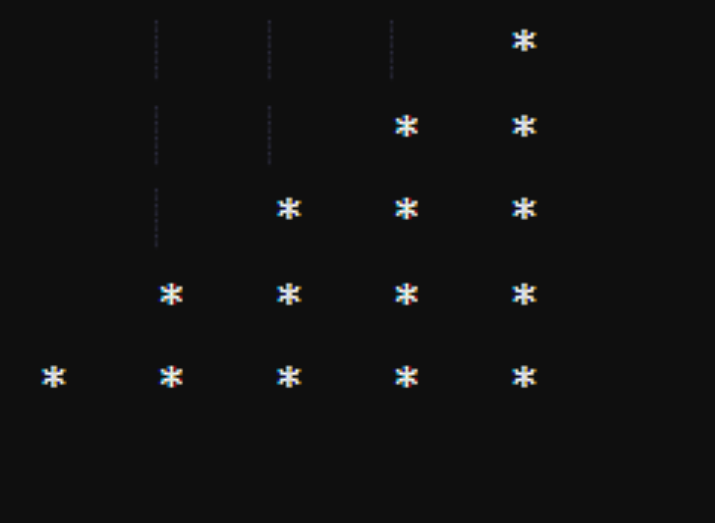### Approach

• Declare and initialize an integer ‘n’ that specifies number of rows.

• Define nested for loop, the outer one will run till ‘n’ and the first inner loop will print the space and the second one will print the stars.

### Example

public class P5 {
public static void main(String[] args) {
int n = 5;
for(int i = 1; i <= n; i++){
for(int spc = 1; spc <= n-i; spc++) {
System.out.print("\t");
}
for(int str = 1; str <= i; str++) {
System.out.print("*\t");
}
System.out.println();
}
}
}


### Output

				*
*	*
*	*	*
*	*	*	*
*	*	*	*	*


## Conclusion

In this article, we have discussed problems on interesting star patterns. These pattern solutions will help you to decode the logic of the pattern questions and make you capable of solving other patterns on your own.

Updated on: 16-May-2023

205 Views